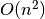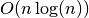# _divide_et_impera¶

`Cartesian.``_divide_et_impera`(maximum_edge_length=25.0, difference_edge=6.0)[source]

Returns a molecule split into cuboids.

If your algorithm scales with. You can use this function as a preprocessing step to make it

scaling with.
Parameters: maximum_edge_length (float) – Maximum length of one edge a cuboid. difference_edge (of) – A dictionary mapping from a 3 tuple of integers to a 2 tuple of sets. The 3 tuple gives the integer numbered coordinates of the cuboids. The first set contains the indices of atoms lying in the cube with a maximum edge length of `maximum_edge_length`. They are pairwise disjunct and are referred to as small cuboids. The second set contains the indices of atoms lying in the cube with ```maximum_edge_length + difference_edge```. They are a bit larger than the small cuboids and overlap with `difference_edge / 2`. dict